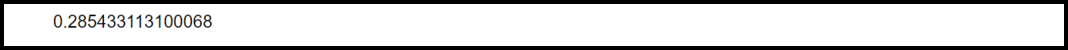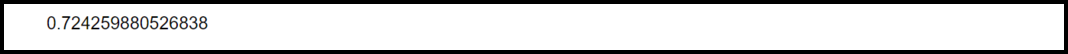Open in App
Not now

# How to Calculate the P-Value of a Chi-Square Statistic in R

• Last Updated : 18 Mar, 2022

Chi-Square Statistic is a method to represent the relationship between two categorical variables. In statistics, variables are categorized into two classes: numerical variables and non-numerical variables (categorical). Chi-square statistic is used to signify how much difference exists between the observed count and the count that is anticipated if there doesn’t exist any relationship in the population. When the Chi-Square test is conducted we get test statistics and with the help of the test statistic, we can find the p-value on the basis of which we can determine whether the test results are statistically significant.

R provides us the pchisq() function using which we can find the p-value of a Chi-Square Statistic. The syntax of this function is given below:

Syntax:

pchisq(q = “value”, df = “value”, lower.tail = TRUE)

Parameters:

• q: It represent the Chi-Square test statistic
• df: It represents the degrees of freedom
• lower.tail = “TRUE”: The probability in the left of q in the Chi-Square distribution is returned.
• lower.tail = “FALSE”: The probability in the right of q in the Chi-Square distribution is returned.

Note that by default lower.tail is TRUE.

## Method 1: Chi-Square Goodness of Fit Test

A salon shop owner proposed that an equal number of customers visit his shop on weekends as well as on weekdays. In order to examine this hypothesis, researchers tracked the number of customers visiting his shop during a week. They find the below information:

Step 1: Hypothesis:

We will now conduct the Chi-Square goodness of fit test that uses the following hypotheses:

• H0: An equal number of customers come into the salon shop each day.
• H1: An equal number of customers do not come into the salon shop each day.

Step 2: Compute the value of (O-E)2 / E for each day.

In total, 70 customers visited the salon shop during the week. Therefore, if we consider that the equal number of people visited his shop each day then the expected value “E” (for each day) comes out to be equal to 10.

Step 3: Calculate the test statistic X2.

X2 = Σ(O – E)2 / E = 0.4 + 1.6 + 0 + 0.4 + 0.9 + 1.6 + 2.5 = 7.4

Step 4: Calculate the p-value of the test statistic X2.

Now let’s calculate the p-value of the test statistic. The q is equal to 7.4 and df is equal to 6.

Example:

## R

 `# Determine the p-value for the Chi-Square test statistic ` `pchisq``(q=7.4, df=6, lower.tail=``FALSE``)`

Output:output

Hence, the p-value associated with X2 = 7.4 and n-1 = 7-1 = 6 degrees of freedom is 0.28543311.

The p-value comes out to be equal to 0.28. Since this value is not less than 0.05. Hence, we would fail to reject the null hypothesis. This implies that we do not have sufficient proof to claim that the actual distribution of the customers differs from the distribution that the owner of the shop proposed.

## Method  2: Chi-Square Test of Independence

Let us consider an example in which researchers are interested to know whether a particular age group preference is associated with soap products.  Two age groups are present in the population:

• Below 18 years.
• Equal to or greater than 18 years.

A random sample of 100 citizens and a survey took place on their soap product preference. Chi-Square Test of Independence was performed and the following information was extracted:

• Chi-Square Test Statistic (X2): 0.64521
• Degrees of freedom: (df): 2

Now we will determine the p-value associated with this Chi-Square test statistic and degrees of freedom.

## R

 `# Determine p-value for the Chi-Square  ` `# test statistic ` `pchisq``(q=0.64521, df=2, lower.tail=``FALSE``)`

Output:Output

The p-value comes out to be equal to 0.72425. Since the p-value is greater than 0.05, hence we cannot follow the null hypothesis. This implies that we do not have sufficient proof to say that there is a link between age group and soap product preference.

My Personal Notes arrow_drop_up
Related Articles